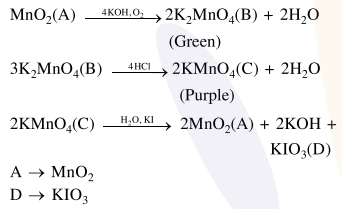Deepak Scored 45->99%ile with Bounce Back Crack Course. You can do it too!

# Prove the following

Question:

$\underline{\mathrm{A}} \stackrel{4 \mathrm{KOH}, \mathrm{O}_{2}}{\longrightarrow} 2 \underline{\mathrm{B}}+2 \mathrm{H}_{2} \mathrm{O}$

$3 \underline{\mathrm{B}} \stackrel{4 \mathrm{HCl}}{\longrightarrow} \underset{(\text { Purple) }}{2} \underline{\mathrm{C}}+\mathrm{MnO}_{2}+2 \mathrm{H}_{2} \mathrm{O}$

$2 \underline{\mathrm{B}} \stackrel{\mathrm{H}_{2} \mathrm{O}, \mathrm{KI}}{\longrightarrow} 2 \underline{\mathrm{A}}+2 \mathrm{KOH}+\underline{\mathrm{D}}$

In the above sequence of reactions,

$\underline{\mathrm{A}}$ and $\underline{\mathrm{D}}$ respectively, are :-

1. $\mathrm{KIO}_{3}$ and $\mathrm{MnO}_{2}$

2. $\mathrm{KI}$ and $\mathrm{K}_{2} \mathrm{MnO}_{4}$

3. $\mathrm{MnO}_{2}$ and $\mathrm{KIO}_{3}$

4. $\mathrm{KI}$ and $\mathrm{KMnO}_{4}$

Correct Option: 3,

Solution: